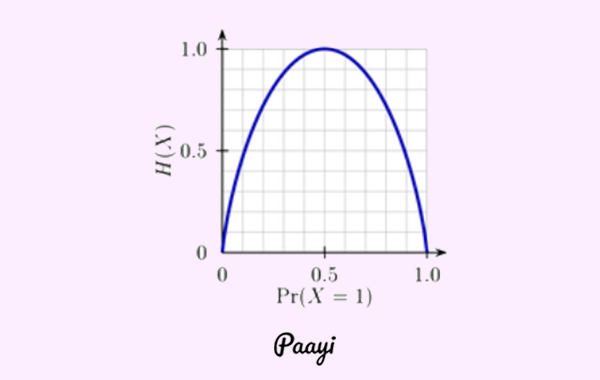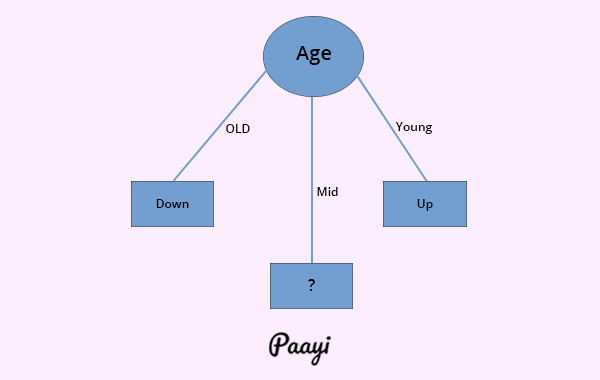## How to Implement Decision Tree Algorithm in Python

##### In this part of Learning Python we Cover Decision Tree Algorithm In Python
Written by |14-May-2019 | 0 Comments | 1351 Views

Entropy:

To define information gain precisely, we begin by defining a measure commonly used in gain information theory known as entropy, that characterizes the extent of impurity in a given set.

Mathematically we can represent entropy:

Entropy(Class) = -( P/P+N ) log2 ( P/P+N ) - ( N/P+N ) log2 ( N/P+N )

where P is a positive number of features and N is the negative number of features.

After finding the entropy of class, we will find the entropy of attributes with a similar formula.

Entropy(Attribute) = -( Pi/P+N ) log2 ( Pi/P+N ) - ( Ni/P+N ) log2 ( Ni/P+N )

where Pi and Ni is the positive and negative occurrence of that attribute and P and N is of class.

To find the information gain

Information Gain = Entropy(Class) – Entropy(Attribute)

The attribute having the maximum gain will be the root node, and this process will continue.Figure 1
• If the dataset contains all 0 or all 1 than Entropy=0
• If the number of Yes = number of No than Entropy= 0.5

Example of Selecting Root Node:

 Age Competition Type Profit old yes software down old no software down old no hardware down mid yes software down mid yes hardware down mid no hardware up mid no software up young yes software up young no hardware up young no software up

Suppose we have a data set give above in which the class is Profit is an attribute and age, competition and type are its feature attributes.

So now we have to calculate what will be the root node for classifying tree. We have to calculate the entropy of the given data set by the formulas which are given above. First, we have to calculate the gain information and then the entropy the attribute with the maximum entropy will be the root node of the tree.

Calculation:

# of Up’s = P =  5            # of Down = N = 5

Entropy(Class) = -( P/P+N ) log2 ( P/P+N ) - ( N/P+N ) log2 ( N/P+N )

by putting the values in an equation we get:

Entropy(class) = 1

Entropy(Age) = 0.4

Gain(Age ) = 1-0.4 = 0.6

Entropy(Competition) = 0.8754

Gain( Competition ) = 1-0.8754 = 0.1245

Entropy(Type) = 1

Gain(Age ) = 1-1 = 0

Information Gain(Age) = 0.6

Information Gain(Competition) = 0.1245

Information Gain(Type) = 0

So the maximum information gain is of age so it will be the root node:Figure 2

And for mid, it will again calculate the entropy.

How to address Overfitting in Decision Trees:

To encounter a problem of overfitting in a decision tree algorithm, the technique that has been used is known as Pruning.

Pruning:

The process of adjusting the tree to minimize the miss-classification of a decision tree is called pruning.

There are two types of pruning:

• Pre Pruning
• Post Pruning

Pre Pruning:

Pre-pruning is the halting of subtree construction at some node after checking some measures. These measures can be information gain, Gini index, etc. If splitting a tree falls below the specific threshold, then pruning is done. It can stop the growth process prematurely.

Post Pruning:

In post pruning, the decision tree grows to its end and after the complete tree is build pruning trim the nodes by making the nodes to the leaf in a bottom-up fashion is done. If error improves after trimming the nodes are replaced by the leaf.

Comparison:

• Pre-pruning is faster than post pruning because later have to wait until the whole tree is built.
• However, post pruning is more effective than pre-pruning as it alters the nodes to leaf after “interaction effect”. These are the effects which arise after several interactions with the attributes.

Stop Criteria of Decision Tree:

• Several cases in the nodes are statistically insignificant.
• If all record in current data subsets has the same output, then don’t recurse.
• If all the records have precisely the same set of input attributes, then don’t recurse.
• If all attributes have zero information gain, then don’t recurse.

Types of Decision Tree:

• ID3
• CART

ID3:

The algorithm creates a multi-way tree. Each node can contain either 2 or more than 2 edges. Then it will find the discrete feature in a dataset that will maximize the information gain by using criterion entropy. It is only appropriate for the classification problem.

• Understandable prediction rules
• build the fast tree-based
• build short tree
• Finding leaf nodes enable test data to be pruned, reducing the number of tests
• Whole Data set is searched to create a tree.

• Data maybe over-fitted or over classified
• Only one attribute at a time is tested for making decisions
• Does not handle numeric attribute and missing values.

CART:

CART stands for Classification and Regression Trees. The algorithm creates a binary tree. Each node has exactly two outgoing edges, finding the best numerical or categorical feature to split using an appropriate impurity criterion. For classification, GINI impurity and entropy method could be used for. CART can also be used for regression by introducing the variance reduction which is based on the least square method.

• It can handle continuous and discrete data.
• This method calculates the feature importance and uses only those features
• It either removes or changes the value of outlier to handle them.

• CART may have an unstable decision tree.
• CART split one by one variable.

• It is simple to understand, translate and visualize using graphs
• Decision tree chooses the best feature by calculating the feature importance.
• Can handle both continuous and discrete data. Can also handle multiple outputs of target variable problems.
• If a user is naive in data preprocessing than decision tree is good because it needs lest input of the user.
• The nonlinear relationship between attribute does not affect tree performance.

• Decision tree learners can create an over-complex tree that does not generalize the data well. It is called over-fitting.
• Decision trees can be unstable because small changes in data might output different
• Decision tree learners can be biased and can give importance to one feature than others.